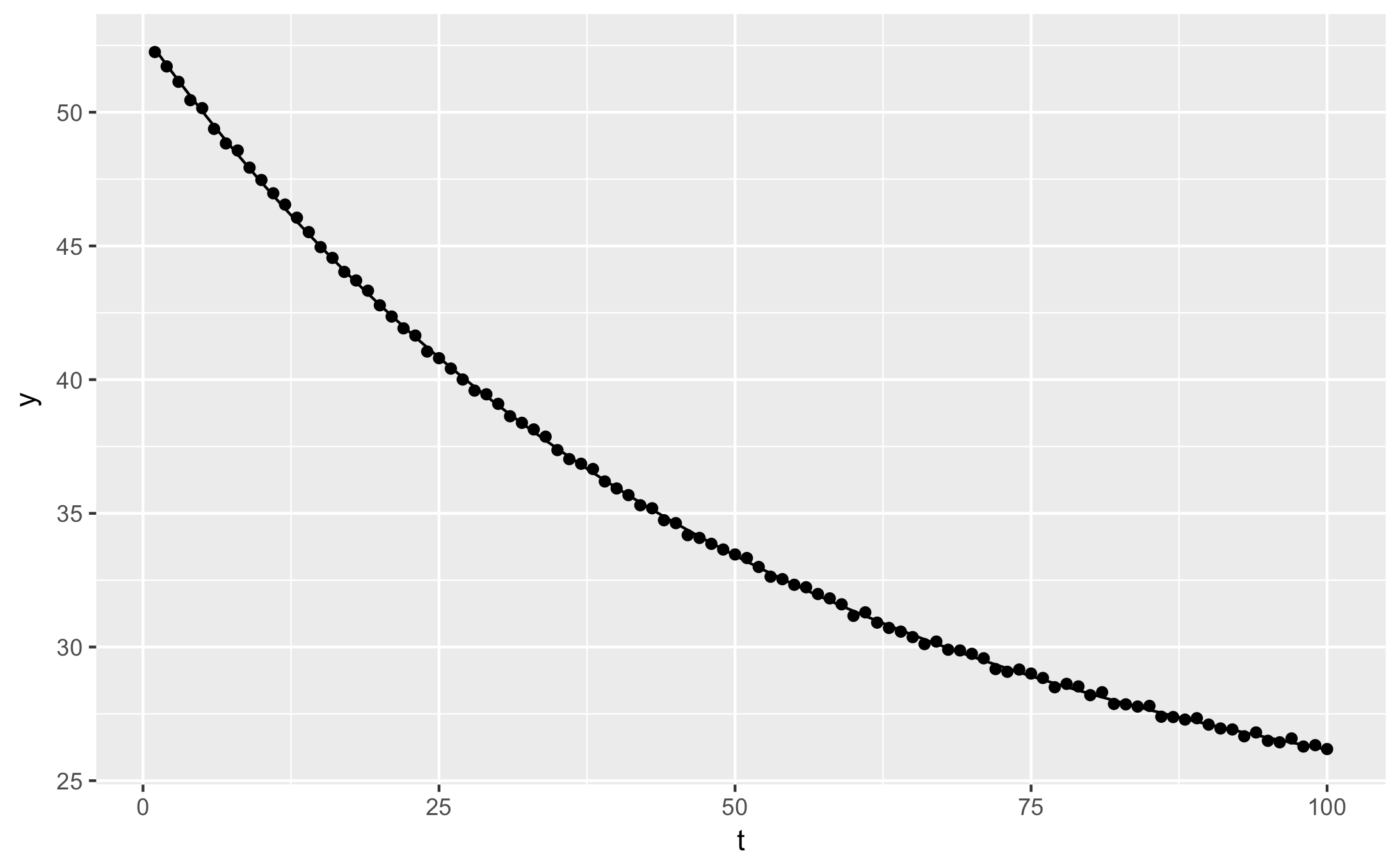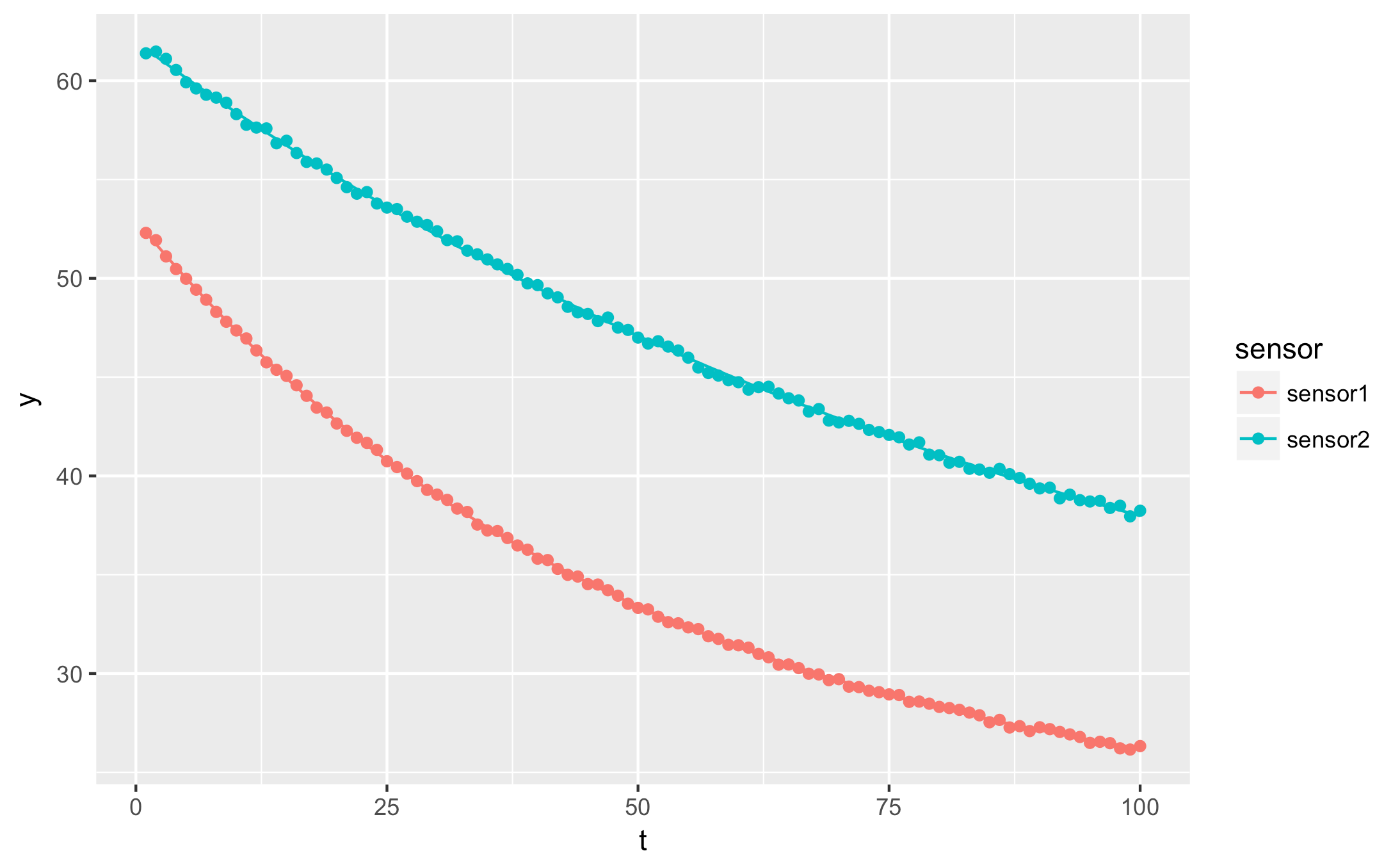Want to share your content on R-bloggers? click here if you have a blog, or here if you don't.

Updated in May 2020 to show a full example with qplot.

Exponential decays can describe many physical phenomena: capacitor discharge, temperature of a billet during cooling, kinetics of first order chemical reactions, radioactive decay, and so on. They are very useful functions, but can be tricky to fit in R: you’ll quickly run into a “singular gradient” error. Thankfully, self-starting functions provide an easy and automatic fix. Read on to learn how to use them.

The formula I’ll use in the following examples is:
$$y(t) \sim y_f + (y_0 – y_f) e^{-\alpha t}$$

The measured value $y$ starts at $y_0$ and decays towards $y_f$ at a rate $\alpha$. Let’s generate some artificial data so you can replicate the examples:

library(tidyverse)
library(broom)

t = 1:100
y1 = 22 + (53 - 22) * exp(-0.02 * t) %>% jitter(10)
y2 = 24 + (60 - 22) * exp(-0.01 * t) %>% jitter(10)

df <- data.frame(t = t, y = y1, sensor = 'sensor1') %>%
rbind(. , data.frame(t = t, y = y2, sensor = 'sensor2'))
sensor1 <- df %>% filter(sensor == 'sensor1')


Our data looks like this:

qplot(t, y, data = df, colour = sensor)## Fitting with NLS

nls is the standard R base function to fit non-linear equations. Trying to fit the exponential decay with nls however leads to sadness and disappointment if you pick a bad initial guess for the rate constant ($\alpha$). This code:

nls(y ~ yf + (y0 - yf) * exp(-alpha * t),
data = sensor1,
start = list(y0 = 54, yf = 25, alpha = 1))


fails with:

Error in nls(y ~ yf + (y0 - yf) * exp(-alpha * t), data = sensor1, start = list(y0 = 54,  :


## Using SSasymp

The solution is to use a self-starting function, a special function for curve fitting that guesses its own start parameters. The asymptotic regression function, SSasymp is equivalent to our exponential decay:

> fit <- nls(y ~ SSasymp(t, yf, y0, log_alpha), data = sensor1)
> fit
Nonlinear regression model
model: y ~ SSasymp(t, yf, y0, log_alpha)
data: sensor1
yf        y0 log_alpha
21.884    52.976    -3.921
residual sum-of-squares: 0.9205

Number of iterations to convergence: 0
Achieved convergence tolerance: 8.788e-07


Its formula is a little different from ours, instead of fitting the rate constant $\alpha$ directly:
$$y(t) \sim y_f + (y_0 – y_f) e^{-\alpha t}$$

It searches for the logarithm of $\alpha$:

$$y(t) \sim y_f + (y_0 – y_f) e^{-\exp(\log\alpha) t}$$

From the fit result, you can plot the fitted curve, or extract whichever other information you need:

qplot(t, y, data = augment(fit)) + geom_line(aes(y = .fitted))For a single curve, it’s easy to guess the approximate fit parameters by looking at the plot, or just trying several values. When fitting many curves however, it is quite convenient to automate the process. Self-starting functions are especially useful combined with dplyr, to fit several experimental conditions in one step.

Here is how we can read out the fit parameters for each sensor in our example data:

df %>%
group_by(sensor) %>%
do(fit = nls(y ~ SSasymp(t, yf, y0, log_alpha), data = .)) %>%
tidy(fit) %>%
select(sensor, term, estimate) %>%
mutate(alpha = exp(log_alpha))

sensor log_alpha y0 yf alpha
sensor1 -3.920671 52.97573 21.88404 0.019827795
sensor2 -4.611703 61.98803 23.85592 0.009934881

Now we know at one glance the rate constant for each sensor location, or the $y$ value that each position will stabilise at.

## Plotting with gglot2

To show both fitted curves on the original data, use broom’s augment function:

augmented <- df %>%
group_by(sensor) %>%
do(fit = nls(y ~ SSasymp(t, yf, y0, log_alpha), data = .)) %>%
augment(fit)

qplot(t, y, data = augmented, geom = 'point', colour = sensor) +
geom_line(aes(y=.fitted))
ggsave("ggplot_exponential_fit.png")augment also yields the residuals. For more ideas on how to apply curve fitting with dplyr, check out my previous article on dplyr.